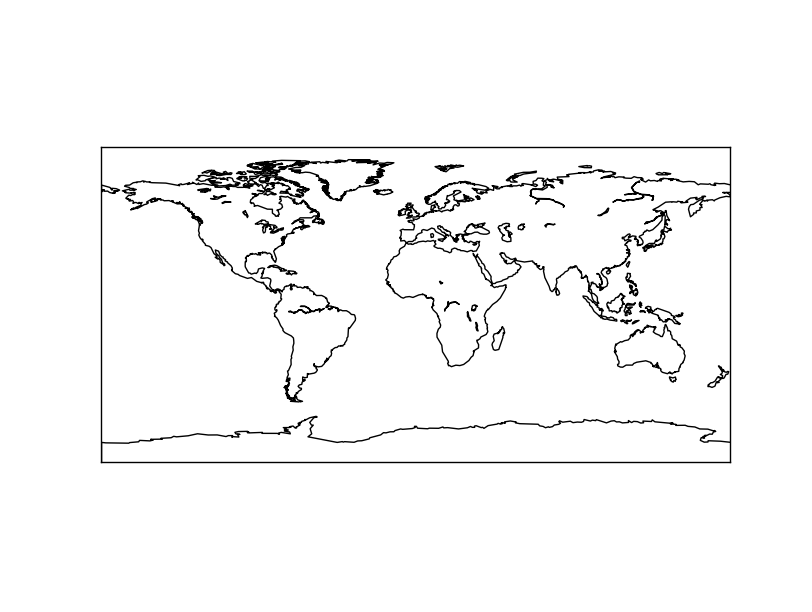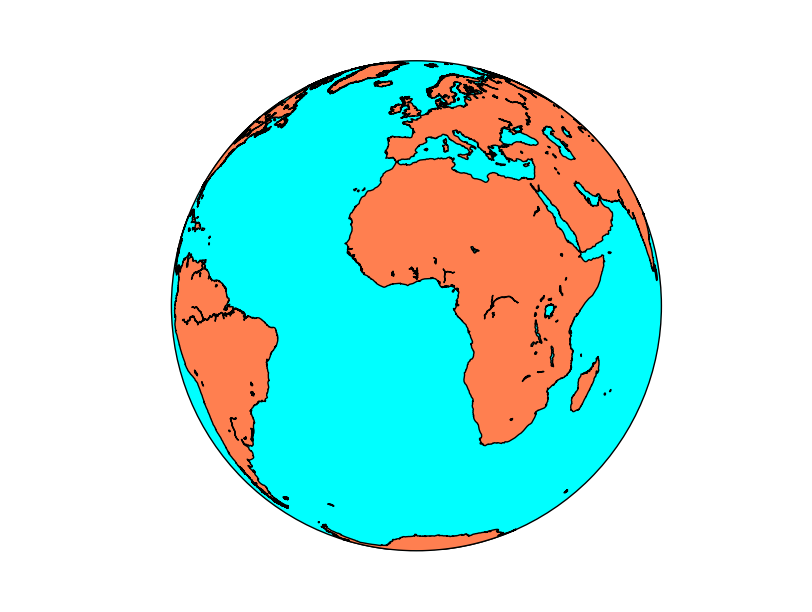# Drawing the first map¶

Let’s create a the simplest map:

```from mpl_toolkits.basemap import Basemap
import matplotlib.pyplot as plt

map = Basemap()

map.drawcoastlines()

plt.show()
plt.savefig('test.png')
```
1. The first two lines include the Basemap library and matplotlib. Both are necessary
2. The map is created using the Basemap class, which has many options. Without passing any option, the map has the Plate Carrée projection centered at longitude and latitude = 0
3. After setting the map, we can draw what we want. In this case, the coast lines layer, which comes already with the library, using the method drawcoastlines()
4. Finally, the map has to be shown or saved. The methods from mathplotlib are used. In this example, plt.show() opens a window to explore the result. plt.savefig(‘file_name’) would save the map into an image.Changing the projection is easy, just add the projection argument and lat_0 and lon_0 to the Basemap contructor.

Even with the new projection, the map is still a bit poor, so let’s fill the oceans and continents with some colors The methods fillcontinents() and drawmapboundary() will do it:

```from mpl_toolkits.basemap import Basemap
import matplotlib.pyplot as plt

map = Basemap(projection='ortho',
lat_0=0, lon_0=0)

#Fill the globe with a blue color
map.drawmapboundary(fill_color='aqua')
#Fill the continents with the land color
map.fillcontinents(color='coral',lake_color='aqua')

map.drawcoastlines()

plt.show()

```